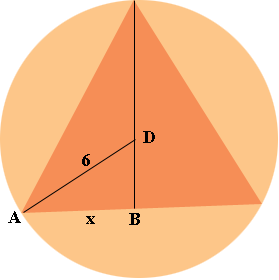SEARCH HOMEMath Central Quandaries & QueriesQuestion from anna, a student: An equilateral triangle is inscribed in a circle of radius 6. Find x and the length of one side of the equilateral triangle. The picture is a triangle where the corners touch the sides of a circle and there is a line drawn down the middle of the triangle. A point labeled D which is in the triangle but I'm pretty sure that its marking the radius of the circle. From that point D is a line going from that point to the bottom left corner of the triangle. So this line shall make another mini triangle. The bottom of the big triangle is then split into 2 segments and the left segment is labeled x. Please help for I am stuck!Hi Anna,

I hope my diagram is the same as yours. I labeled two additional points.Angle ABD is a right angle. Can you see why?

The measure of angle DAB is 30o. Can you see why?

Triangle DAB is then a 30-60-90 triangle. What do you know about the side length of a 30-60-90 triangle?

I hope this helps. If not, write back,
PennyMath Central is supported by the University of Regina and The Pacific Institute for the Mathematical Sciences.